# What Are The Output Values Of Tangent? (5 Key Values To Know)

In trigonometry, you will sometimes see common input angles (such as 45 or 90 degrees) for the tangent function.  It is helpful to become familiar with the corresponding outputs for these angles so you can do calculations faster.

So, what are the output values of tangent?  Five output values of tangent you should know are tan(0) = 0, tan(π/6) = √3/3, tan(π/4) = 1, tan(π/3) = √3, and tan(π/2) is undefined. These output values correspond to the common degree measures 0o, 30o, 45o, 60o, and 90o. Using the negative of these angles as input gives us the negative of the above outputs.

Of course, when the input angle for tangent is in quadrants 2 or 4 (angles between 90o and 180o, or π/2 to 3π/2 radians, and 270o and 360o, or 3π/2 to 2π radians), we get negative values for tangent.  When the input angle for tangent is in quadrants 1 or 3 (angles between 0o and 90o or 180o and 270o), we get positive values for tangent.

In this article, we’ll talk about some output values for tangent and how to remember the 5 common ones we listed above.  We’ll also review the signs of the outputs for tangent and when they are positive, negative, or zero.

Let’s get started.

## What Are The Output Values Of Tangent?

The output values of tangent are given in the table below for 5 common values:

There are a couple of different ways to remember or derive these values.

One way is to remember that the tangent of an angle A is the same as the sine of the angle A divided by the cosine of the angle A, or:

• tan(A) = sin(A)/cos(A)

This is because of SOH-CAH-TOA: tangent is opposite over adjacent, and sine is opposite over hypotenuse, while cosine is adjacent over hypotenuse.

So, for example:

• tan(60) = sin(60) / cos(60) = (√3/2) / (1/2) = √3

As another way to derive values, remember that tan(90 – A) is equal to 1/tan(A). This is because of the complementary sine and cosine identities, which state that:

• sin(90 – A) = cos(A)
• cos(90 – A) = sin(A)

Using both of the above identities, we can prove that:

• tan(90 – A)
• =sin(90 – A) / cos(90 – A)
• =cos(A) / sin(A)
• = 1/tan(A)

Remember that we measure reference angles from the x-axis to get an acute angle (less than 90 degrees).  This means that in the 2nd quadrant, we subtract the angle from 180 degrees (π radians) to get our reference angle.

Also, keep in mind that the output values of tangent are negative in the 2nd quadrant.

So, in the 2nd quadrant, we would get the following reference angles and output values for tangent:

In the 3rd quadrant, we subtract 180 degrees (π radians) from the angle to get our reference angle.

Also, the output values of tangent are positive in the 3rd quadrant (since both sine and cosine are negative in the 3rd quadrant, so their ratio is positive).

So, in the 3rd quadrant, we would get the following reference angles and output values for tangent:

In the 4th quadrant, we subtract the angle from 360 degrees (2π radians) to get our reference angle.

Also, the output values of tangent are negative in the 4th quadrant.

In the 4th quadrant, we would get the following reference angles and output values for tangent:

Finally, remember that for a negative angle, we add 360 degrees (2π radians) until we get to an angle between 0o and 360o.

For an angle greater than 360o, we do the opposite and subtract 360 degrees (2π radians) until we get to an angle between 0o and 360o.

### Examples Of Output Values Of Tangent

Let’s take a look at some examples to show how to find the output values of tangent for various input angles.

#### Example 1: Tangent In The First Quadrant

Let’s say that we have the angle A = 60o, which is in the first quadrant (it is between 0o and 90o).

To find the tangent of the angle A, all we need to do is look up the value in the first table above.

So:

• tan(A)
• =tan(60o)
• =√3

#### Example 2: Tangent In The Second Quadrant

Let’s say that we have the angle A = 150o, which is in the second quadrant (it is between 90o and 180o).

To find the tangent of the angle A, we will first need to find the reference angle A’.  In the second quadrant, we calculate

• A’ = 180o – A
• A’ = 180o – 150o
• A’ = 30o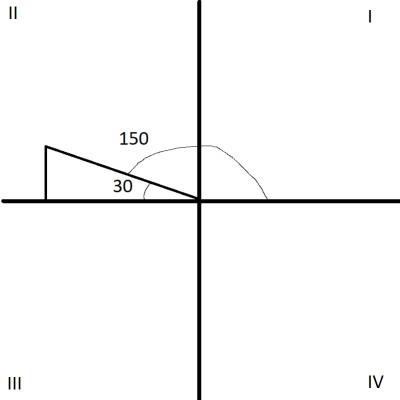A 150 degree angle is in the second quadrant (quadrant II). We can look up the output value for the reference angle (30 degrees) in a tangent table.

Also, we know that tangent has negative output in the 2nd quadrant.  Now, we need to look up the value in the first table above.

So:

• tan(A)
• =tan(150o)
• =-tan(30o)  [using our reference angle, A’ = 30o, and tangent is negative in the 2nd quadrant]
• =-√3/3  [tan(30o) = √3/3, from the table above]

#### Example 3: Tangent In The Third Quadrant

Let’s say that we have the angle A = 225o, which is in the third quadrant (it is between 180o and 270o).

To find the tangent of the angle A, we will first need to find the reference angle A’.  In the third quadrant, we calculate

• A’ = A – 180o
• A’ = 225o – 180o
• A’ = 45o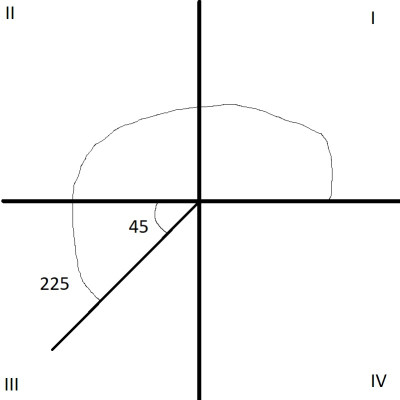A 225 degree angle is in the third quadrant (quadrant III). We can look up the value of the reference angle (45 degrees) in a tangent table.

Also, we know that tangent has positive output in the 3rd quadrant.  Now, we need to look up the value in the first table above.

So:

• tan(A)
• =tan(225o)
• =tan(45o)  [using our reference angle, A’ = 45o, and tangent is positive in the 3rd quadrant]
• =1  [tan(45o) = 1, from the table above]

#### Example 4: Tangent In The Fourth Quadrant

Let’s say that we have the angle A = 330o, which is in the fourth quadrant (it is between 270o and 360o).

To find the tangent of the angle A, we will first need to find the reference angle A’.  In the fourth quadrant, we calculate

• A’ = 180o – A
• A’ = 360o – 330o
• A’ = 30o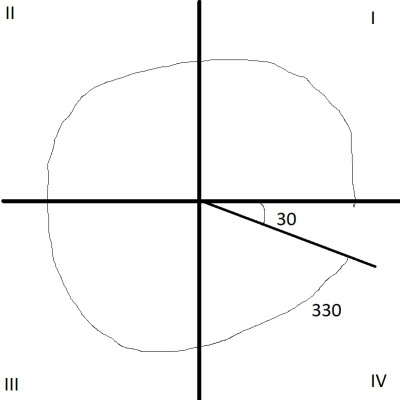A 330 degree angle is in the fourth quadrant (quadrant IV). We can look up the value of the reference angle (30 degrees) in a tangent table.

Also, we know that tangent has negative output in the 4th quadrant.  Now, we need to look up the value in the first table above.

So:

• tan(A)
• =tan(330o)
• =-tan(30o)  [using our reference angle, A’ = 30o, and tangent is negative in the 4th quadrant]
• =-√3/3  [tan(30o) = √3/3, from the table above]

#### Example 5: Tangent Of A Negative Angle

Let’s say that we have the angle A = -150o, which is negative.  So, we will add 360o until we get an angle measure between 0o and 360o.

This gives us B = -150o + 360o = 210o.

To find the tangent of the angle A = -150o, we can find the tangent of angle B = 210o (note that B = 210o is in the 3rd quadrant, since it is between 180o and 270o).

First, we need to find the reference angle B’.  In the 3rd quadrant, we calculate

• B’ = B – 180o
• B’ = 210o – 180o
• B’ = 30o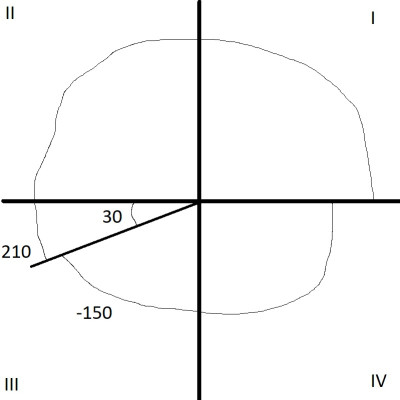A -150 degree angle is in the third quadrant (quadrant III), and is equivalent to an angle of 210 degrees. We can look up the value of the reference angle (30 degrees) in a tangent table.

Also, we know that tangent has positive output in the 3rd quadrant.  Now, we need to look up the value in the first table above.

So:

• tan(A)
• =tan(B)
• =tan(210o)
• =tan(30o)  [using our reference angle, B’ = 30o, and tangent is positive in the 3rd quadrant]
• =√3/3  [tan(30o) = √3/3, from the table above]

#### Example 6: Tangent Of An Angle Greater Than 360o

Let’s say that we have the angle A = 630o, which is greater than 360o.  So, we will subtract 360o until we get an angle measure between 0o and 360o.

This gives us B = 630o – 360o = 270o.

To find the tangent of the angle A = 630o, we can find the tangent of angle B = 270o (note that B = 270o is on the border between the 3rd and 4th quadrants, since 270o is the cutoff).

First, we need to find the reference angle B’.  We calculate

• B’ = B – 180o
• B’ = 270o – 180o
• B’ = 90o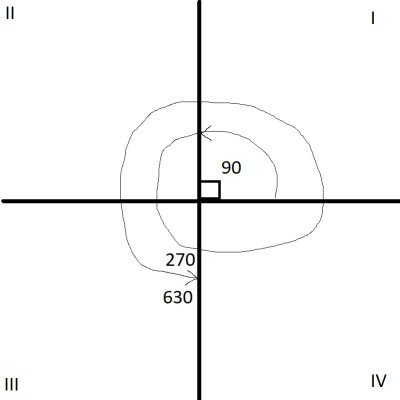A 630 degree angle is in the third quadrant (quadrant III), and is equivalent to an angle of 270 degrees. We can look up the value of the reference angle (90 degrees) in a tangent table.

Also, we know that tangent has positive output in the 3rd quadrant.  Now, we need to look up the value in the first table above.

So:

• tan(A)
• =tan(B)
• =tan(270o)
• =tan(90o)  [using our reference angle, B’ = 90o, and tangent is positive in the 3rd quadrant]
• =undefined  [tan(90o) is undefined, from the table above]

## Conclusion

Now you know more about the output values of tangent and how they change as input angles change.  You also know how to figure out the sign of the outputs, based on the quadrant of the input angle.